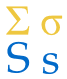# 总和符号

Σ   这符号 （叫 总和符号，英语：Sigma） 的意思是 "加起来"

Σ 的意思是"加起来"……

Σ
n
所以在这里是加 n

### 但 n 的值是多少？

n 的值是显示在总和符号的

4
Σ
n=1
n
这个的意思是n 从 1 到 4，

### 好，来算……

4
Σ
n=1
n = 1 + 2 + 3 + 4 = 10## 更强大

Σ 可以做更强大的运算！

4
Σ
n=1
n2 = 12 + 22 + 32 + 42 = 30

4
Σ
n=1
(2n+1) = 3 + 5 + 7 + 9 = 24

3
Σ
i=1
i(i+1) = 1×2 + 2×3 + 3×4 = 20

5
Σ
i=3
i i + 1 = 34 + 45 + 56

## 为什么英语叫 "Sigma""Sigma" 是希腊语的大写字母 S，而S 代表英语字 "Sum"（和）。